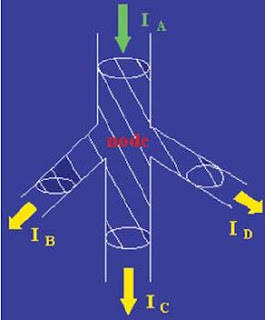KIRCHHOFF'S LAWS

## KIRCHHOFF’S LAWS

As the network becomes complex, the application of Ohm’s law for solving the networks becomes tedious and hence time-consuming. For solving such complex networks, we make use of Kirchhoff’s laws. Gustav Kirchhoff (1824-1887), an eminent German physicist, did a considerable amount of work on the principles governing the behavior of electric circuits. He gave his findings in a set of two laws: (i) current law and (ii) voltage law, which together are known as Kirchhoff’s laws.

KIRCHHOFF’S CURRENT LAW

The first law is Kirchhoff’s current law (KCL), which states that the algebraic sum of currents entering any node is zero.

Let us consider the node shown in Figure 1. The sum of the currents entering the node is

-ia+ib-ic+id=0

Or

ia-ib+ic-id=0

Which simply states that the algebraic sum of currents leaving a node is zero. Alternatively, we can write the equation as

ib+id=ia+ic

Which states that the sum of currents entering a node is equal to the sum of currents leaving the node. If the sum of the currents entering a node were not equal to zero, then the charge would be accumulating at a node. However, a node is a perfect conductor and cannot accumulate or store charge. Thus, the sum of currents entering a node is equal to zero.

KIRCHHOFF’S VOLTAGE LAW

Kirchhoff’s voltage law (KVL) states that the algebraic sum of voltages around any closed path in a circuit is zero.

In general, the mathematical representation of Kirchhoff’s voltage law is

∑Nj=1Vj(t)=0

Where Vj(t) is the voltage across the jth branch (with proper reference direction) in a loop containing N voltages.

In Kirchhoff’s voltage law, the algebraic sign is used to keep track of the voltage polarity.

In other words, as we traverse the circuit, it is necessary to sum the increases and decreases in voltages to zero. Therefore, it is important to keep track of whether the voltage is increasing or decreasing as we go through each element. We will adopt a policy of considering the increase in voltage as negative and a decrease in voltage as positive.

Consider the circuit shown in Figure 2, where the voltage for each element is identified with its sign. The ideal wire used for connecting the components has zero resistance, and thus the voltage across it is equal to zero. The sum of voltages around the loop incorporating V6, V3, V4, V5 is

−V6−V3+V4+V5=0

The sum of voltages around a loop is equal to zero. A circuit loop is a conservative system, meaning that the work required to move a unit charge around any loop is zero.

However, it is important to note that not all electrical systems are conservative. Example of a non-conservative system is a radio wave broadcasting system.

## IN OTHER WORDS OF KIRCHHOFF’S LAWS

The statements of Kirchhoff’s laws are shown in Art. For DC networks instead of an algebraic sum of currents and voltages, we take phasors or vector sums for AC networks.PIN IT

1. KIRCHHOFF’S CURRENT LAW:

According to this law, in any electrical network, the phasors sum of the currents meeting at a junction is zero.

In other words, ∑ I = 0 —– at a junction.

Put in another way, it simply means that in any electrical circuit, the phasors sum of the currents flowing towards a junction is equal to the phasors sum of the currents going away from that junction.

2. KIRCHHOFF’S VOLTAGE LAW:

According to this law, the phasors sum of the voltage drops across each of the conductors in any closed path (or mesh) in a network plus the phasors sum of the EMFs connected in that path is zero.

In other words, ∑ IR + ∑ emf = 0 —- round a mesh.